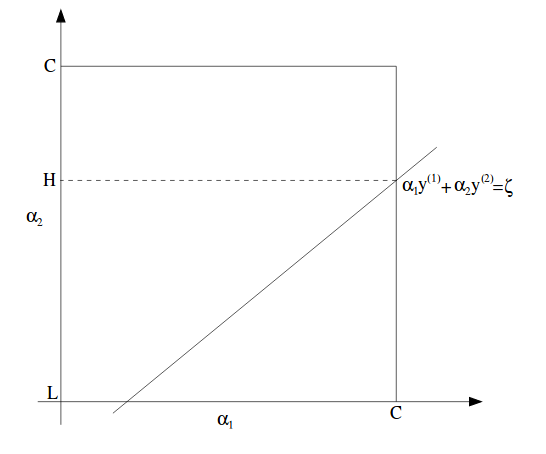# Support Vector Machine Chinese Version

Please note this post is a study note translated to Chinese by me. Click here to see the original English version in Wei’s homepage.

# 支持向量机

## 1 直观理解与符号应用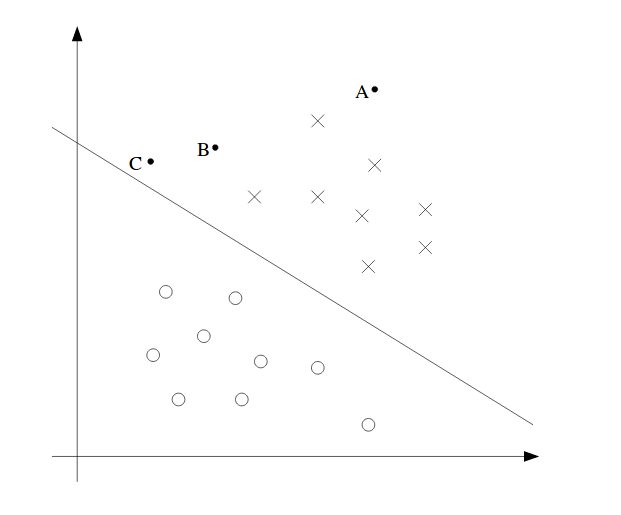## 2 函数间隔与几何间隔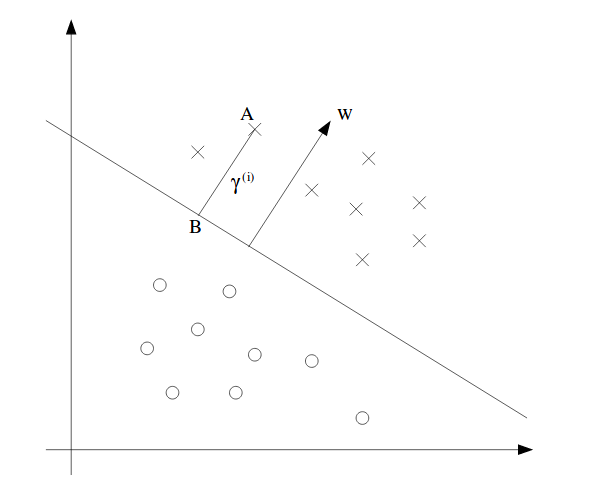$x_i-x_j$是沿着边界线的向量。我们知道如果两个向量的点积为零，那么这两个向量是相互垂直的。所以$w^T$与$(x_i-x_j)$互相垂直。

## 4 拉格朗日对偶性

Primal 和 dual problem 的相关性为:

## 5 OMC与拉格朗日对偶性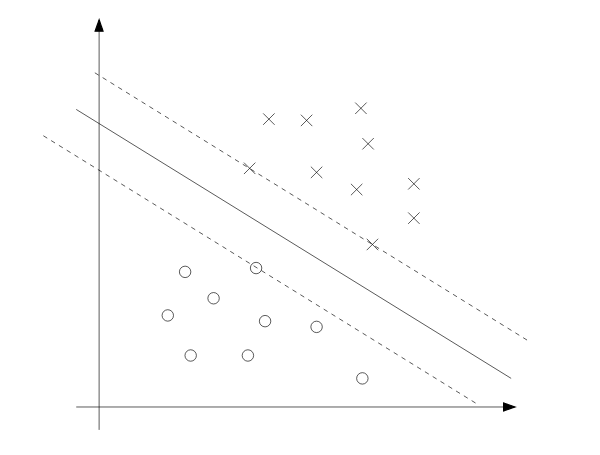## 6 核函数

(1)对称矩阵: $K_{ij} = K(x^{(i)},x^{(j)}) = \phi(x^{(i)})^T\phi(x^{(j)}) = \phi(x^{(j)})^T\phi(x^{(i)}) = K_{ji}$

(2)半正定矩阵: $z^TKz \geq 0$ 这个证明很简单，如果需要的话可以提供过程。

Mercer定理（Mercer Theorem的中文无对应翻译）：设$K:\mathbb{R}^n \times \mathbb{R}^n \mapsto \mathbb{R}$。为了使核函数有效，对于任何${x^{(1)},\dots,x^{(m)}}$，对应的核矩阵必须同时是对称的，也是半正定的，这是一个充要条件。

## 7 正规化与无法分割问题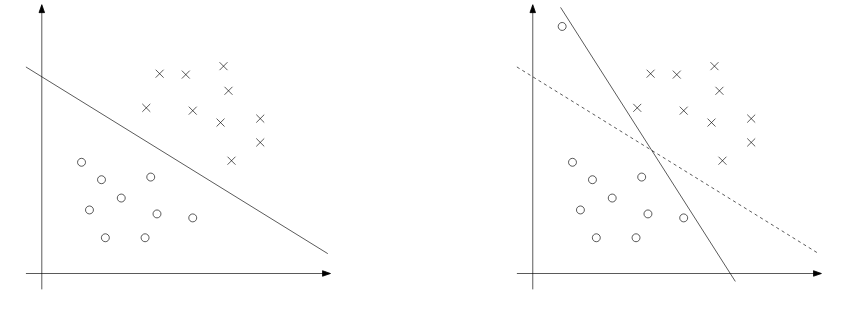(1) 我们希望w小，这样间隔就会大。

(2) 我们希望大多数样本的函数间隔大于1。

## 8 序列最小优化算法（SMO）

John Platt的SMO（顺序最小优化）算法的出现是用来解决SVM中的dual problem的。

### 8.1 坐标上升法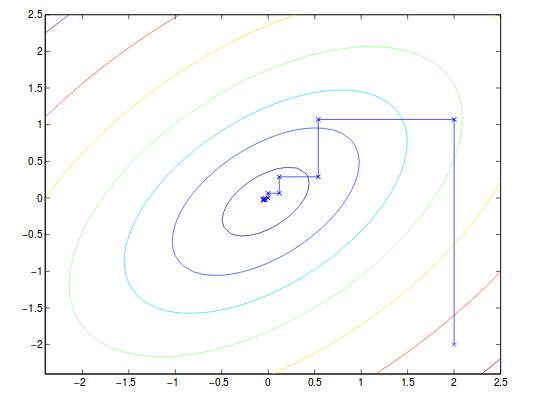### 8.2 序列最小优化算法（SMO）